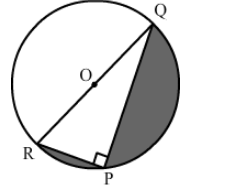# In the given figure, PQ = 24, PR = 7 cm and O is the centre of the circle.`
Question:

In the given figure, PQ = 24, PR = 7 cm and O is the centre of the circle. Find the area of the shaded region.Solution:

In the right ">ΔRPQ, we have:

$R Q=\sqrt{R P^{2}+P Q^{2}}$

$=\sqrt{7^{2}+24^{2}}$

$=\sqrt{49+576}$

$=25 \mathrm{~cm}$

OR OQ = 12.5 cm
Now,

Area of the circle $=\pi r^{2}$

$=3.14 \times 12.5 \times 12.5$

$=490.625$ sq. $\mathrm{cm}$

Area of the semicircle $=\frac{490.625}{2}=245.31$ sq. $\mathrm{cm}$

Area of the triangle $=\frac{1}{2} \times b \times h=\frac{1}{2} \times 7 \times 24=84$ sq. $\mathrm{cm}$

Thus, we have:

Area of the shaded part = Area of the semicircle $-$ Area of the triangle

$=245.31-84$

$=161.31 \mathrm{~cm}^{2}$